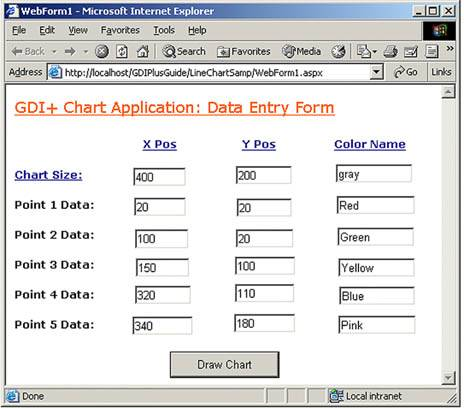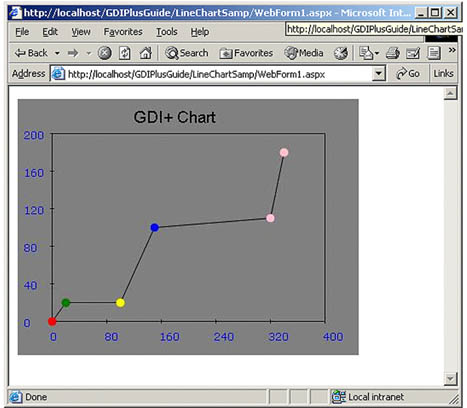# Drawing a Line Chart

Charts are useful for representing numeric data in a graphical way. There are several different types of charts, including pie, line, and bar charts. In this section we will learn how to use GDI+ and ASP.NET to draw a line chart from data entered by a user.

A line chart is a set of continuous lines. In the example presented in this section, we will read the size of the chart and data points and draw a chart based on the points. Our discussion will focus first on the ChartComp component, and then on the client application.

12.5.1 The ChartComp Component

ChartComp is a class that defines the functionality to add points to the chart and draw the chart. The client application (discussed in Section 12.5.2) is a Web application that calls the chart's members to add points to the chart and draw it.

The code for the ChartComp class is given in Listing 12.8. The constructor of the class takes the type, color, size, and a page to which this chart belongs. The overloaded InsertPoint method adds a point to the array of points, and the DrawChart method draws the points stored in the array. DrawChart first draws a rectangle, and then it draws points toward the x- and y-axes.

Listing 12.8 The ChartComp class

```// Chart component
class ChartComp
{
public Bitmap curBitmap;
public ArrayList ptsArrayList =
new ArrayList();
public float X0 = 0, Y0 = 0;
public float chartX, chartY;
public Color chartColor = Color.Gray;
// chartType: 1=Line, 2=Pie, 3=Bar.
// For future use only.
public int chartType = 1;
private int Width, Height;
private Graphics g;
private Page curPage;
struct ptStructure
{
public float x;
public float y;
public Color clr;
}
// ChartComp constructor
public ChartComp(int cType, Color cColor,
int cWidth, int cHeight, Page cPage)
{
Width = cWidth;
Height = cHeight;
chartX = cWidth;
chartY = cHeight;
curPage = cPage;
chartType = cType;
chartColor = cColor;
curBitmap = new Bitmap(Width, Height);
g = Graphics.FromImage(curBitmap);
}
// Destructor. Disposes of objects.
~ChartComp()
{
curBitmap.Dispose();
g.Dispose();
}
// InsertPoint method. Adds a point
// to the array.
public void InsertPoint(int xPos,
int yPos, Color clr)
{
ptStructure pt;
pt.x = xPos;
pt.y = yPos;
pt.clr = clr;
// Add the point to the array
}
public void InsertPoint(int position,
int xPos, int yPos, Color clr)
{
ptStructure pt;
pt.x = xPos;
pt.y = yPos;
pt.clr = clr;
// Add the point to the array
ptsArrayList.Insert(position, pt);
}
// Draw methods
public void DrawChart()
{
int i;
float x, y, x0, y0;
curPage.Response.ContentType="image/jpeg";
g.SmoothingMode = SmoothingMode.HighQuality;
g.FillRectangle(new SolidBrush(chartColor),
0, 0, Width, Height);
int chWidth = Width-80;
int chHeight = Height-80;
g.DrawRectangle(Pens.Black,
40, 40, chWidth, chHeight);
g.DrawString("GDI+ Chart", new Font("arial",14),
Brushes.Black, Width/3, 10);
// Draw x- and y-axis line, points, positions
for(i=0; i<=5; i++)
{
x = 40+(i*chWidth)/5;
y = chHeight+40;
string str = (X0 + (chartX*i/5)).ToString();
g.DrawString(str, new Font("Verdana",10),
Brushes.Blue, x-4, y+10);
g.DrawLine(Pens.Black, x, y+2, x, y-2);
}
for(i=0; i<=5; i++)
{
x = 40;
y = chHeight+40-(i*chHeight/5);
string str = (Y0 + (chartY*i/5)).ToString();
g.DrawString(str, new Font("Verdana",10),
Brushes.Blue, 5, y-6);
g.DrawLine(Pens.Black, x+2, y, x-2, y);
}
// Transform coordinates so that point (0,0)
// is in the lower left corner
g.RotateTransform(180);
g.TranslateTransform(-40, 40);
g.ScaleTransform(-1, 1);
g.TranslateTransform(0, -(Height));
// Draw all points from the array
ptStructure prevPoint = new ptStructure();
foreach(ptStructure pt in ptsArrayList)
{
x0 = chWidth*(prevPoint.x-X0)/chartX;
y0 = chHeight*(prevPoint.y-Y0)/chartY;
x = chWidth*(pt.x-X0)/chartX;
y = chHeight*(pt.y-Y0)/chartY;
g.DrawLine(Pens.Black, x0, y0, x, y);
g.FillEllipse(new SolidBrush(pt.clr),
x0-5, y0-5, 10, 10);
g.FillEllipse(new SolidBrush(pt.clr),
x-5, y-5, 10, 10);
prevPoint = pt;
}
curBitmap.Save(curPage.Response.OutputStream,
ImageFormat.Jpeg);
}
}
```

12.5.2 The Client Application

The client application is a Web page that is used to get input from the user. The main form of the application is shown in Figure 12.14. The user can enter his/her chart size, and values for five points, including the color of each one.

Figure 12.14. Entering points on a chartThe Draw Chart button draws a line chart. Code for the Draw Chart button click is given in Listing 12.9, where we create an object of type ChartComp and call its InsertPoint and DrawChart methods. InsertPoint adds a point to the chart. DrawChart draws a line chart from the first point to the last point entered by the user.

Listing 12.9 The Draw Chart button click event handler

```private void Button1_Click(object sender,
System.EventArgs e)
{
// Get the chart background color
Color clr = Color.FromName(TextBox3.Text);
// Create a ChartComp object
ChartComp chart =
new ChartComp(1, clr, 400, 300, this.Page);
chart.X0 = 0;
chart.Y0= 0;
chart.chartX = Convert.ToInt16(TextBox1.Text);
chart.chartY = Convert.ToInt16(TextBox2.Text);
// Add points to the chart
chart.InsertPoint(Convert.ToInt16(TextBox4.Text),
Convert.ToInt16(TextBox5.Text),
Color.FromName(TextBox6.Text) );
chart.InsertPoint(Convert.ToInt16(TextBox7.Text),
Convert.ToInt16(TextBox8.Text),
Color.FromName(TextBox9.Text) );
chart.InsertPoint(Convert.ToInt16(TextBox10.Text),
Convert.ToInt16(TextBox11.Text),
Color.FromName(TextBox12.Text) );
chart.InsertPoint(Convert.ToInt16(TextBox13.Text),
Convert.ToInt16(TextBox14.Text),
Color.FromName(TextBox15.Text) );
chart.InsertPoint(Convert.ToInt16(TextBox16.Text),
Convert.ToInt16(TextBox17.Text),
Color.FromName(TextBox18.Text) );
// Draw chart
chart.DrawChart();
}
```

Now if you use the data entered in Figure 12.14 and click the Draw Chart button, the output will look like Figure 12.15.

Figure 12.15. A line chart in ASP.NETGDI+ Programming with C#
ISBN: 073561265X
EAN: N/A
Year: 2003
Pages: 145